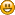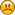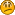## Weapon Employment Zone

Started by 375CT, June 06, 2014, 12:17:55 AM

#### 375CT

Hi there,

I'd assume most have read about the WEZ analysis Litz is now including as a feature in its PC software.

Would like to hear about opinions on its value as "hit probability" tool, if it's such, and mostly about the math behind it, if it makes sense. So far, I was only aware of Montecarlo simulations but apparently this feaure is not based off of it.

Any comments are greatly appreciated.

#1
WEZ is a Monte Carlo simulation.

I find it hard to opinate about it - it does what it says.

Quite frequently I use a similar tool understand ballistic effects in a target shoot competition setting.

It is available as download on the BfX site. Look for the descriptive text:

"This workbook hosts a simulator with which you can estimate your results in a (multi distance, multi target) match, e.g. the NRA 90 shot full bore regional match course."

#### 375CT

#2
Quote from: admin on June 11, 2014, 01:16:00 AM
WEZ is a Monte Carlo simulation.

I find it hard to opinate about it - it does what it says.

Quite frequently I use a similar tool understand ballistic effects in a target shoot competition setting.

It is available as download on the BfX site. Look for the descriptive text:

"This workbook hosts a simulator with which you can estimate your results in a (multi distance, multi target) match, e.g. the NRA 90 shot full bore regional match course."

Robert,

Thanks for the answer, however how do you know WEZ is based off of Montecarlo? I've read all the available literature by Litz and that word is not present even once.

thanks!

#3
Maybe "Monte Carlo" is a  definition issue. In this case, i imagined that input parameters are generated in such a way that they are consistent with a certain probability distribution. Then a trajectory calculated and evaluated. The process is repeated hundreds of times. This is what one might describe as Monte Carlo.

#### 375CT

#4
Quote from: admin on June 12, 2014, 01:38:37 PM
Maybe "Monte Carlo" is a  definition issue. In this case, i imagined that input parameters are generated in such a way that they are consistent with a certain probability distribution. Then a trajectory calculated and evaluated. The process is repeated hundreds of times. This is what one might describe as Monte Carlo.

Nice reply, much clear now. Will take a look to your workbook and for sure coming back with more questions, if you don't mind#5
Ok,
I understand what you understand - nothing special meant here. Let me make things less abstract.

Basically what Monte Carlo does is to generate "outcomes", e.g. where a  bullet ends, for, varying conditions - the input parameters. The input parameters are varied according to a certain probability distribution. If for example a shooter is able to aim with an accuracy of 1 MoA then people like me model that as a bell-shaped (Gaussian) probability distribution that averages to 1 MoA mean inaccuracy. With this we acknowledge the fact that in some cases the shooter aims very well and in others badly.

In a Monte Carlo simulation then, the computer draws a random number and converts that to an inital horizontal and vertical aiming angle. This is done in such a way that average inaccuracy is 1 MoA. After the horizontal and vertical launch angles are chosen, the bullets trajectory is calculated.

If, for instance, in addition the effects of a non-constant wind is being studied, one might model that primitively as a wind distribution that is half of the time zero and the other half 1 m/s. In a Monte Carlo calculation then one first draws a random number that determines the launch angle, and an another random number is used to determine if there is wind or not in the trajectory calculation (with the afforementioned spreadsheet I deal on a more sophisticated way with non constant wind along a trajectory). Hence we end up with a set of bullet end coordinates that  were affected by both wind and the shooters abilities.

In practise, the more inputs one generates according to a probability distribution, the less the details of a probability distribution matters. The distribution might as well be flat, meaning that the probability for a certain input value is constant in a certain range and zero elsewhere.

#### mman

#6
See Hit probability thread as well. My tool is based on analytical formulas. I have compared the results with litz's tool and the difference is usually less than 5%. I have done some assumptions that are not mathematically exact. However it's still pretty close. In theory benefit is that calculation time is reduced significantly compared to monte carlo method. However I'm not an expert in programming and my code is not as effective as robert's. My program also includes more variables. That's why theoretical benefit in calculation time is not redeemed in practice.

The limitation also is that I can only calculate round targets while with monte carlo target shape is not limited.

Here is as an article about circular probability error which I have used. It is also far better measure for gun accuracy than extreme spread when it comes to probability of hitting something.

Robert, how many iterations you need to do with monte carlo before you get hit probability say in accuracy of 1%? 99 % of the time?

#### 375CT

#7
Excellente discussion and insight!Just a simple question, since I'm not that familiar with Excel programming (just the basics), do you think that using a random number generator (RNG), for using a common programming language, could do the same? I mean, assuming that the RNG is based on a Gaussian distribution?

On the other hand, what other kind of probability distribution do you guys thinks is worth exploring for a realistic scenario?

I find this subject very interesting for developing some sort of BfX simulator, besides the excellent work already done by Robert.

Mman, if you don't me asking, why you state your work is not mathematically exact?

#### mman

#8
I'll let robert answer the programming stuff...

If you look into commercial programs gaussian distribution is the most common for gun accuracy. To apply anything else you would need really extensive testing and fitting.

I have done a shooting diary as well (see Group and velocity statistics thread) and I have now around 500 groups in my database. When it comes to statistics it's not much but it is something. In avarage all the real world group measures I have saved fit very well to gaussian distribution. So I would say it really is pretty good probability distribution for the application.

If you accept that holes distribute according to gaussian distribution you can calculate lot's of things. For example I have done extensive testing with monte carlo comparing different group size measures. There are lots of them. To mention few: radial extreme spread (the most common and worst), ES X and Y directions, radial standard deviation, SD X and Y directions, diagonal, Circular error probability (which is best I have found). There are at least two important things which define the best measure: 1. effectivenes which means that how many rounds you have to shoot for two different loads to define which is more accurate. 2. independence which means how number of shots or group shape affects to measure in question.

The best feature in SD based group measures is that they are not depended on number of shots in the group like extreme spread measures. For example with monte carlo you can calculate that 10-shot group is around 1,6x (if my memory serves me right) larger than 3-shot group. And that's only true if groups are round in average. While CEP is in average approx. the same for both 3- or 10-shot groups.  If group ellipcity factor is not higher than 4.

Quote from: 375CT on June 24, 2014, 07:29:08 AM
Mman, if you don't me asking, why you state your work is not mathematically exact?
Read the article about CEP. Derivation includes few approximations at least on ellipcity and bias. Then again I have assumed that all the dispersion factors are independed. This is pretty good approximation but not exactly true as roberts states on Hit probability thread.

#9
In visual basic and as an Excel function I have published random number generators, two of them generate numbers with a constant probability ("flat").
The other one. =bfx_rang(....) generates numbers according to a gaussian distribution.
The properties of Excels random generators did not suit my purpose.

The "flat" distribution is the basis for generating numbers distributed according to another distribution.
For gaussian distributions efficient algorithms can be googled.

Nevertheless, the more random variables involved in a simulation, the less details of a certain random variable matter.

#### mman

#10
What's wrong with excel's RAND ( ) ? My monte carlo is based on that and I got same averages as some references I used...

#### 375CT

#11
Quote from: admin on June 25, 2014, 01:47:55 PM
In visual basic and as an Excel function I have published random number generators, two of them generate numbers with a constant probability ("flat").
The other one. =bfx_rang(....) generates numbers according to a gaussian distribution.
The properties of Excels random generators did not suit my purpose.

The "flat" distribution is the basis for generating numbers distributed according to another distribution.
For gaussian distributions efficient algorithms can be googled.

Nevertheless, the more random variables involved in a simulation, the less details of a certain random variable matter.

Understood the last part, but it's not clear why RANDOM in VB is not good enough. I got the point on Gaussian but I read somewhere that random() is indeed gaussian, so more confussion for me at least#### mman

#12
http://office.microsoft.com/en-gb/excel-help/rand-function-HP010342816.aspx
This is flat. You can convert it into gaussian if you want.

There is discussion about it:

There seems to be an agreement what robert said about it. It would be interesting to hear why and how bad rand() actually is.

#### 375CT

#13
Confirmed here

http://msdn.microsoft.com/en-us/library/2dx6wyd4(v=vs.110).aspx

Random in VB.net yields an uniform distribution...now, I need to figure out how bad this could be for creating something akin a WEZ run.

Anyway, like Robert pointed out, a RNG can be easily programmed if such need ever arises with for instance the Ziggurat algorithm.

Mman, thanks for the links, they just confirmed my suspicions on this too.

Now will also review CEP. Well, so far the original questions remains, is Litz's WEZ (a term taken from Air Combat for budget error analysis) flat or not?#### mman

#14
Quote from: 375CT on June 26, 2014, 11:44:10 PM
Well, so far the original questions remains, is Litz's WEZ (a term taken from Air Combat for budget error analysis) flat or not?That's easy one. It must be normal distribution. No sense to use flat distribution, that would lead to strange results.